189 信息 1045 成员

## Forager 是如何管理成千上百个实例的### 绘制优化

• Primitives (`draw_rectangle, draw_circle, draw_primitive_begin, etc.`)
• Surfaces (`surface_set_target, surface_reset_target`)
• Shaders (`shader_set_target, shader_reset_target`)
• Draw settings (`draw_set_font, draw_set_colour, etc.`)
• GPU settings (`gpu_set_blendmode, gpu_set_alphaenable, etc.`)

``````// Outline
draw_self();

``````// Instance outlines
with (par_outlined) {
draw_self();
}

### 步（Step）事件优化

``````if (1 + 1 == 3) && (instance_place(x, y, obj_enemy)) {
// stuff
}``````

### 更进一步：动态加载实例### 一些实际代码### 真·实例 函数### 再快一点### 结语（转发自：原日志地址
##### GAMEMAKER STUDIO 2中实现2D动态光影效果（PART 2）``surf = -1 ;``

``````if( !surface_exists(surf) ){
surf = surface_create(room_width,room_height);
}``````

``````surface_set_target(surf);
draw_clear_alpha(0,0);

vertex_begin(VBuffer, VertexFormat);
for(var yy=starty;yy<=endy;yy++)
{
for(var xx=startx;xx<=endx;xx++)
{
}
}
vertex_end(VBuffer);
vertex_submit(VBuffer,pr_trianglelist,-1);
surface_reset_target();

draw_surface(surf,0,0);````````````shader_set(shader0);
draw_surface(surf,0,0);

``uniform vec4 u_fLightPositionRadius;        // x=lightx, y=lighty, z=light radius, w=unused``

``````varying vec2 v_vTexcoord;
varying vec4 v_vColour;
varying vec2 v_vScreenPos;``````

``v_vScreenPos =  vec2( in_Position.x, in_Position.y );``

``LightPosRadius = shader_get_uniform(shader0,"u_fLightPositionRadius");``

``````shader_set(shader0);
draw_surface(surf,0,0);

``````varying vec2 v_vTexcoord;
varying vec4 v_vColour;
varying vec2 v_vScreenPos;

void main()
{
// Work out vector from room location to the light

// work out the length of this vector
float dist = sqrt(vect.x*vect.x + vect.y*vect.y);

// if in range use the shadow texture, if not it's black.
gl_FragColor = v_vColour * texture2D( gm_BaseTexture, v_vTexcoord );
}else{
gl_FragColor = vec4(0.0, 0.0, 0.0, 1.0);
}
}````````gl_FragColor.a *= 0.5 ;````vec2 vect = v_vScreenPos.xy-u_fLightPositionRadius.xy;``

``````//在范围内使用阴影纹理，否则全黑。
// 从光源中央往半径将值从 0 变为 1
// 获得阴影纹理
gl_FragColor = v_vColour * texture2D( gm_BaseTexture, v_vTexcoord );
// 阴影线性变为全黑
gl_FragColor = mix( gl_FragColor, vec4(0.0,0.0,0.0,0.7), falloff);
}else{
// 超出半径的直接全黑
gl_FragColor = vec4(0.0, 0.0, 0.0, 0.7);
}````````draw_surface_ext(surf,0,0,1,1,0,image_blend,0.5);``

``````    vec4 col =  texture2D( gm_BaseTexture, v_vTexcoord );
if( col.a<=0.01 ){
gl_FragColor = v_vColour;
}else{
gl_FragColor = col;
}``````（转发自：原日志地址
##### GameMaker Studio 2中实现2D动态光影效果（Part 1）

——————————```var lx = mouse_x;       // 光源坐标，基于鼠标的位置
var ly = mouse_y;
var rad = 96            // 发光半径
var tile_size = 32;     // 瓷片的尺寸
var tilemap = layer_tilemap_get_id("walls");    //获取摆放墙的 tilemap id

//定位待检测矩形区域顶点坐标

//绘制黄色矩形区域
draw_set_color(c_yellow);
draw_rectangle(startx*tile_size,starty*tile_size, endx*tile_size,endy*tile_size,true);  ```

```for(var yy=starty;yy<=endy;yy++)
{
for(var xx=startx;xx<=endx;xx++)
{
var tile = tilemap_get(tilemap,xx,yy);
if( tile!=0 ){
}
}
}```

``````/// @description 初始化阴影构成
vertex_format_begin();
VertexFormat = vertex_format_end();

VBuffer = vertex_create_buffer();````````````vertex_begin(VBuffer, VertexFormat);
for(var yy=starty;yy<=endy;yy++)
{
for(var xx=startx;xx<=endx;xx++)
{
var tile = tilemap_get(tilemap,xx,yy);
if( tile!=0 )
{
// get corners of the
var px1 = xx*tile_size;     // top left
var py1 = yy*tile_size;
var px2 = px1+tile_size;    // bottom right
var py2 = py1+tile_size;

}
}
}
vertex_end(VBuffer);
vertex_submit(VBuffer,pr_trianglelist,-1);``````

``````Adx = PointX-LightX;

``````/// @description 根据光源位置投射某线段的阴影
/// @param VB Vertex buffer
/// @param Ax  x1
/// @param Ay  y1
/// @param Bx  x2
/// @param By  y2
/// @param Lx  Light x
/// @param Ly  Light Y

var _vb = argument0;
var _Ax = argument1;
var _Ay = argument2;
var _Bx = argument3;
var _By = argument4;
var _Lx = argument5;
var _Ly = argument6;

// 阴影是无限的——至少要完全覆盖整个屏幕

// 获取点 1 的单元向量长度

// 获取点 2 的单元向量长度
Bdx = _Bx-_Lx;
Bdy = _By-_Ly;
len = (1.0*SHADOW_LENGTH) / sqrt( (Bdx*Bdx)+(Bdy*Bdy) );    // unit length scaler * Shadow length
Bdx = _Bx + Bdx * len;
Bdy = _By + Bdy * len;

vertex_position(_vb, _Ax,_Ay);
vertex_argb(_vb, \$ff000000);
vertex_position(_vb, _Bx,_By);
vertex_argb(_vb, \$ff000000);
vertex_argb(_vb, \$ff000000);

vertex_position(_vb, _Bx,_By);
vertex_argb(_vb, \$ff000000);
vertex_argb(_vb, \$ff000000);
vertex_position(_vb, Bdx,Bdy);
vertex_argb(_vb, \$ff000000);````````````  if( !SignTest( px1,py1, px2,py1, lx,ly) ){
}
if( !SignTest( px2,py1, px2,py2, lx,ly) ){
}
if( !SignTest( px2,py2, px1,py2, lx,ly) ){
}
if( !SignTest( px1,py2, px1,py1, lx,ly) ){
}``````

``````/// @description 检测点位于线条哪一侧
/// @param Ax
/// @param Ay
/// @param Bx
/// @param By
/// @param Lx
/// @param Ly

var _Ax = argument0;
var _Ay = argument1;
var _Bx = argument2;
var _By = argument3;
var _Lx = argument4;
var _Ly = argument5;

return ((_Bx - _Ax) * (_Ly - _Ay) - (_By - _Ay) * (_Lx - _Ax));``````（转发自：原日志地址
##### GMS2：draw_text_ext小坑一枚

``draw_text(x,y,string)``

``draw_text_ext(x, y, string, sep, w);``

``````/// @func draw_chinese_text_ext(x, y, str, sep, w, less_or_more);
/// @param x_ordinate        x 坐标
/// @param y_ordinate        y 坐标
/// @param string                绘制的字符串内容
/// @param sepration          行间距
/// @param width                 单行最大像素宽度
/// @param l_or_m              单行取大于或小于上述宽度

var xx = argument;
var yy = argument;
var str = argument;
var sep = argument;
var w = argument;
var l = noone;
if (argument_count > 5) l = argument else l = true;

var x0 = xx;
var y0 = yy;

while!(str == ""){
var s = "";
var i = 0;
var ww = 0;
var con = "";
do{
i++;
s = string_copy(str, 1, i);
ww = string_width(s)
con = string_copy(s, i, 1);
}until(ww >= w) or (i >= string_length(str)) or (con == "#");
if (con!="#"){
if (l) and (ww > w){
var char = string_char_at(s, string_length(s));
var t = char == "）" or char == "，" or char == "。" or char == "！" or char == "？";
if !(t){
i--;
s = string_copy(str, 1, i);
}
}
draw_text(x0, y0, s);
y0 += sep;
str = string_delete(str, 1, i);
} else {
s = string_copy(str, 1, i-1);
draw_text(x0, y0, s);
y0+=sep;
str = string_delete(str, 1, i);
}
}
return noone;``````

（转发自：原日志地址

• 站立
• 行走/跑
• 躲闪
• 爬墙

## 认真脸：流程图。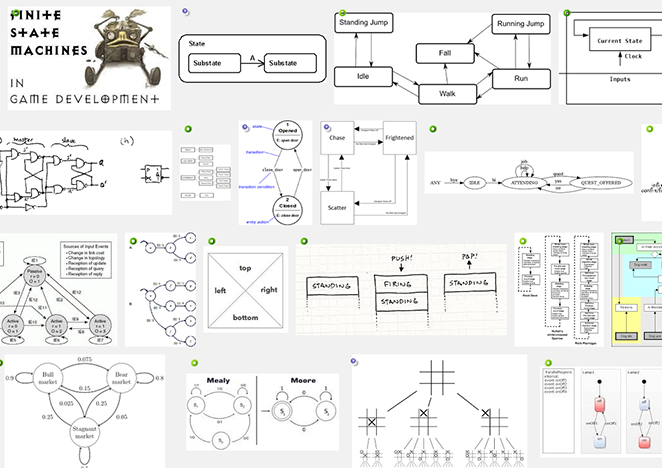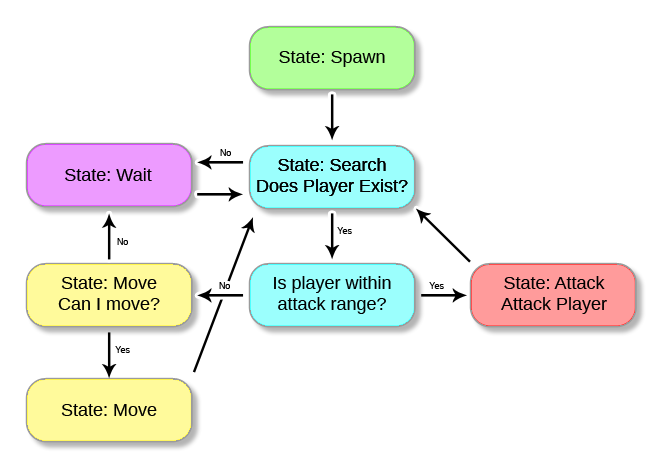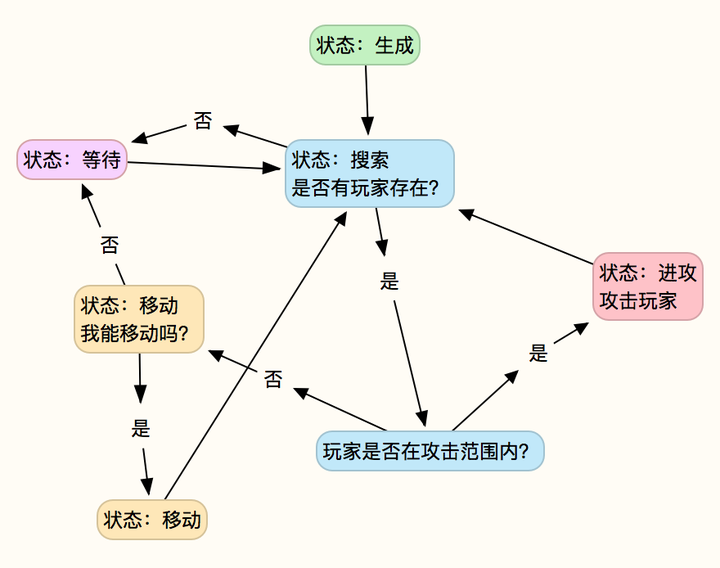## OK，设计好了。怎么实现呢？

state_machine_init()

• state - 这个变量是当前状态的标志位。这个脚本中将会包含在对象step事件中执行的代码。
• state_next - 当我们切换状态时，我们希望在切换之前当前状态能执行完毕，因此我们调用这个脚本来切换状态，同时更新这个值，然后上述"state"变量将在"End Step"事件中发生变化。
• state_name - 这个变量用于获取保存当前状态的名称（创建时设定的名称）
• state_timer - 这个变量用于记录当前状态持续的“step”数（即运行了多少帧），实用程度绝对超乎想象
• state_map - 一个ds_map数据结构，把你设定的状态名称作为键名保存进来，
• state_stack - 一个用来记录你历史状态的数据结构。可以用来实现一些状态机的进阶功能，比如变回到之前的状态。
• state_new - 这是个非常有用的变量，当你切换到某个状态时，有可能你希望该状态处于初始化状态，比如速度设为0，或者更新精灵等等，这些情况十分常见。这时候你只需要在状态的最开始将这个值设为“true”即可完成这一切操作。
• state_var - state_var 这个变量比较特别。这是一个用来存储某个状态的持续时间的数组。因为我发现我自己经常会有这样的需求——“我想要清楚地跟踪并记录这个状态下的一些信息……但是别的状态没这种需求”那我该怎么办？每次有这种需求的时候都新建一个变量？这不是很荒谬嘛？所以，我用“state_var”来作为替代品，把这个数组作为针对该状态的一个便笺本，或是剪贴板，取决于你的用法。这个数组可以记录我所需要的值，因此我不必新建变量来进行记录，可能有点说不太清楚，但这个非常有用。

State_create("state name",state_script)

``````state_create("Stand",state_mario_stand);    //调用"state_mario_stand"脚本处理“站立”状态
state_create("Walk",state_mario_walk);        //调用"state_mario_walk"脚本处理“行走”状态
state_create("Air",state_mario_air);            //调用"state_mario_air"脚本处理“空中”状态
state_create("Crouch",state_mario_crouch);    //调用"state_mario_crouch"脚本处理“蹲下”状态
``````

state_init("State Name")

``````state_init("Stand");     //把“站立”状态设置为默认初始状态
``````

state_execute()

state_update()

state_cleanup()

state_switch("State Name" or state_script)

``````if(keyboard_check_pressed(vk_down))
switch_state("Crouch");
``````

state_switch_previous()

## 尾声

（转发自：原日志地址
##### [ 分享 ]GMS2摄像机系统指南

RUNTIME: 2.0.1.27

# 概要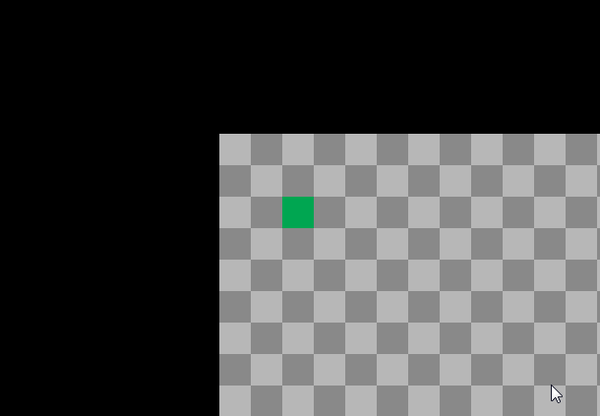# 教程

• Gamemaker Studio 2缩写为GMS2
• 请用GML，英雄！
• 困了惑了，请随时按F1查手册，英雄！
• 发现了错误，或者有什么问题请email我，英雄！（去这里找原作者）
• 译注：视图对应view，摄像机对应camera，视图端口对应viewport，加括号有点恶心……sry，mate

• 设置一个视图（用来使用摄像机）
• 创建/删除摄像机并分配给视图
• 通过代码设置摄像机跟随对象（object）
• 除了绑定视图之外，用其他方法绘制摄像机
• 摄像机脚本 - 更新、开始和结束
• 比较简单的摄像机位置插值
• 比较简单的摄像机缩放
• 根据场景边界限定摄像机位置
• 其余的摄像机函数和功能

## 设置一个视图（用来使用摄像机）

OKay，你可能想跳过这里……我知道，你要是知道这玩意的话就跳过吧。实际上就是在一个Room内，通过编辑器或者代码来启用视图。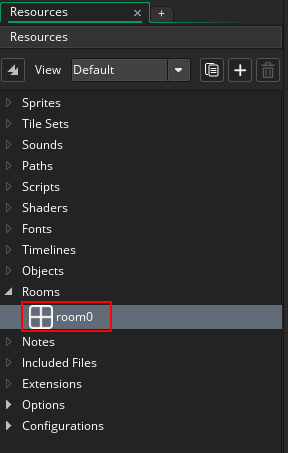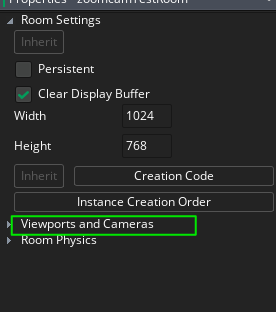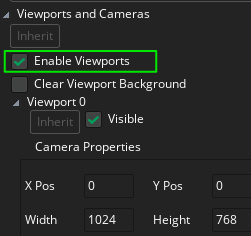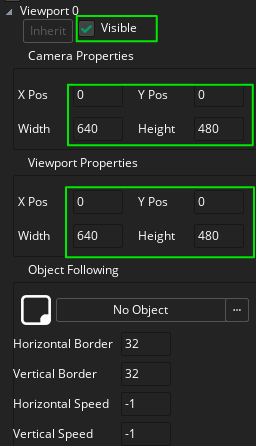OK，我们搞定了基本的视图设置。

• 跟随对象（Object Following）：如字面意思，让摄像机跟随选中的对象。
• 视图边界（View Border）：摄像机跟随对象之前，需要靠近视图边界的距离（以像素为单位）。
• 视图速度（Camera Speed）：当越过边界时，摄像机跟随对象移动的速度。速度设为-1会让视图即时移动来显示跟随对象。

``````//启用视图
view_enabled = true;

//设置view 0为可见
view_set_visible(0, true);

//设置view 0的视图端口尺寸为640x480，边界为0
view_set_wport(0, 640);
view_set_hport(0, 480);``````

``````//调整尺寸并居中
window_set_rectangle((display_get_width() - view_wport) * 0.5, (display_get_height() - view_hport) * 0.5, view_wport, view_hport);
surface_resize(application_surface,view_wport,view_hport);``````

## 创建和删除摄像机并分配给视图

"camera_create()" 和 "camera_create_view()"两个函数都返回唯一的摄像机ID，通过ID我们可以用来使用其他所有的摄像机相关函数。

"camera_create()"用来创建一个实际上是空白画布的摄像机 - 你需要手动指定位置和尺寸，然后才能在2D中正确使用 - 我这样说是因为你可以在3D中使用它而无需指定这些参数。"camera_create_view()"更适合2D开发来使用，因为它强制你在创建时就要指定所有的视图参数。

``````//创建摄像机
//在（0,0）的位置创建一个摄像机，640x480，角度为0，没有跟随实例，摄像机水平和垂直速度为即时跳转，边界为32像素
camera = camera_create_view(0, 0, 640, 480, 0, -1, -1, -1, 32, 32);``````

``camera_destroy(camera);``

``````//设置view0来使用摄像机 "camera"
view_set_camera(0, camera);``````

## 通过代码设置摄像机跟随对象（object）

``````//创建一个摄像机，位置(0,0), 尺寸640x480, 角度0, 跟随的目标实例"objPlayer", 即时跳转的水平垂直速度，边界为32像素
camera = camera_create_view(0, 0, 640, 480, 0, objPlayer, -1, -1, 32, 32);``````

``````//基础设置
camera_set_view_pos(view_camera, 0, 0);
camera_set_view_size(view_camera, 640, 480);

//设置目标对象的信息
camera_set_view_target(objPlayer);
camera_set_view_speed(view_camera, -1, -1);
camera_set_view_border(view_camera, 32, 32);``````

## 除了绑定视图之外，用其他方法绘制摄像机

``````//应用摄像机并以此来绘制
camera_apply(camera);``````

``````if(view_current == 0)
{
camera_apply(camera);
...
}
...``````

``camera_apply(camera_get_default());``

## 摄像机脚本 - 更新、开始和结束

OK，这些函数都可以正常工作，并以类似的方式使用 - 你可以告诉它们什么时候开始工作。在这里，我要将脚本赋予视图0的摄像机的开始函数。

``````//对分配给当前视图的摄像机进行震动
camera_set_view_pos(view_camera[view_current], random_range(-6,6), random_range(-6,6));``````

``````//将很挫的震屏脚本update_script绑定到所需的摄像机"begin"
camera_set_begin_script(view_camera[target_view],update_script);``````

``````camera_set_end_script(view_camera[target_view],update_script);
camera_set_update_script(view_camera[target_view],update_script);``````

OK，脚本内容先聊到这儿。

## 比较简单的摄像机位置插值

``````click_x = 0;
click_y = 0;``````

``````//检测鼠标左键是否点击，是的话，更新点击位置
if(mouse_check_button_pressed(mb_left))
{
click_x = mouse_x;
click_y = mouse_y;
}

//获取目标视图位置和尺寸，尺寸减半所以视图将聚焦在中心
var vpos_x = camera_get_view_x(view_camera);
var vpos_y = camera_get_view_y(view_camera);
var vpos_w = camera_get_view_width(view_camera) * 0.5;
var vpos_h = camera_get_view_height(view_camera) * 0.5;

//插值率
var rate = 0.2;

//将视图位置插入新的对应位置
var new_x = lerp(vpos_x, click_x - vpos_w, rate);
var new_y = lerp(vpos_y, click_y - vpos_h, rate);

//更新视图位置
camera_set_view_pos(view_camera, new_x, new_y);``````

• 我们获取当前位置（即左上角）和视图的尺寸
• 将视图尺寸减半
• 我们建的变量rate，用来指定插值的速率（这样后面改起效果来会方便一些）
• 我们用lerp函数将当前视图的位置定位到新的点击位置，视图的宽高减半会从点击位置移除，让视图聚焦在 中心
• 将新的位置坐标赋予视图，过程结束

## 摄像机缩放

``````zoom_level = 1;
//获取初始视图尺寸，后面要用于插值
default_zoom_width = camera_get_view_width(view_camera);
default_zoom_height = camera_get_view_height(view_camera);``````

``````//根据鼠标滚轮来缩放，用clamp函数可以让效果看起来没那么蠢
zoom_level = clamp(zoom_level + (((mouse_wheel_down() - mouse_wheel_up())) * 0.1), 0.1, 5);

//获取当前视图尺寸
var view_w = camera_get_view_width(view_camera);
var view_h = camera_get_view_height(view_camera);

//设置插值率
var rate = 0.2;

//通过插值计算当前和目标缩放尺寸获取新视图尺寸
var new_w = lerp(view_w, zoom_level * default_zoom_width, rate);
var new_h = lerp(view_h, zoom_level * default_zoom_height, rate);

//应用新尺寸
camera_set_view_size(view_camera, new_w, new_h);``````

• 根据鼠标滚轮当前的滚动方式调整缩放级别 - 向上放大向下缩小。缩放级别也用clamp函数处理，防止过度缩放
• 获取当前的视图尺寸
• 设定插值率（这样后面改起效果来会方便一些）
• 通过当前视图尺寸插入到原始尺寸乘以缩放级别来确定新尺寸的大小
• 更新新的视图尺寸

``````//获取重新对齐视图所需的位移值
var shift_x = camera_get_view_x(view_camera) - (new_w - view_w) * 0.5;
var shift_y = camera_get_view_y(view_camera) - (new_h - view_h) * 0.5;

//更新视图位置
camera_set_view_pos(view_camera,shift_x, shift_y);

## 根据场景边界限定摄像机位置

``````camera_set_view_pos(Camera_ID,
clamp( camera_get_view_x(Camera_ID), 0, room_width - camera_get_view_width(Camera_ID) ),
clamp( camera_get_view_y(Camera_ID), 0, room_height - camera_get_view_height(Camera_ID) ));``````

## 其余的摄像机函数和其功能

• 我们可以用view_set_[x,y,w,h]port()函数来改变视图端口相关的东西
• 可以通过view_set_surface_id()告诉视图绘制到surface
• camera_get_active()返回当前活跃摄像机的唯一ID（正在绘制的摄像机）
• camera_set_proj_mat()采用投影矩阵，通常用三个矩阵函数之一 - matrix_build_projection_ortho，matrix_build_projection_perspective或matrix_build_projection_perspective_fov
• camera_set_view_mat()采用视图矩阵，通常用matrix_build_lookat构建
• 我在另一篇指南“GMS2的3D开发起步”讲了这些矩阵函数的基本用法
• 本指南中讨论的所有setter函数都对应的有getter函数

*译注：阿西吧，真tm长………

（转发自：原日志地址

## 参数说明

argument0 x1 待检测坐标的x
argument1 y1 待检测坐标的y
argument2 x2 需要检测碰撞的瓷片地图ID

## 代码正文

``````///@description tiles_collision(x , y , tilemap)
///@arg x
///@arg y
///@arg tilemap

var xx, yy, tilemap, xp, yp, meeting;

xx = argument0;
yy = argument1;
tilemap = argument2;

//save our current position
xp = x;
yp = y;

//move to the position where we wanna check for a tile collision
x = xx;
y = yy;

//check for collision on all four corners of the collision mask
meeting =       tilemap_get_at_pixel(tilemap, bbox_right, bbox_top)
||
tilemap_get_at_pixel(tilemap, bbox_right, bbox_bottom)
||
tilemap_get_at_pixel(tilemap, bbox_left, bbox_top)
||
tilemap_get_at_pixel(tilemap, bbox_left, bbox_bottom);

//Move back to the original position
x = xp;
y = yp;

//Return wether or not there was a collision
return(meeting);``````

## 使用范例

``````var tile_solid = layer_tilemap_get_id("tiles_ground")
if(!tiles_collision(x, y + 1, tile_solid))
{
speed_down  += gravity_;
}``````

（转发自：原日志地址
##### [ 分享 ]在GMS中绘制技能冷却动画### 简单说明• 可以在调用方法前使用draw_set_alpha和draw_set_colour来定义绘制的不透明度和颜色（用完记得要恢复）
• 原方法绘制了一个矩形，如果你想绘制圆形，可以使用纹理贴图来实现
• 对调x1和x2的传入顺序可以切换顺时针/逆时针动画方向
• 传入的value并不是冷却时间而应当是当前剩余的冷却进度（百分比）

### 代码

``````/// draw_rectangle_cd(x1, y1, x2, y2, value)
///@param x1
///@param y1
///@param x2
///@param y2
///@param value

var v, x1, y1, x2, y2, xm, ym, vd, vx, vy, vl;
v = argument4
if (v <= 0) return 0 // 进度为0则不绘制
x1 = argument0; y1 = argument1; // 定义左上角
x2 = argument2; y2 = argument3; // 定义右下角
if (v >= 1) return draw_rectangle(x1, y1, x2, y2, false) // 填满冷却区域
xm = (x1 + x2) / 2; ym = (y1 + y2) / 2; // 定义中心点
draw_primitive_begin(pr_trianglefan)
draw_vertex(xm, ym); draw_vertex(xm, y1)
// 绘制不同的角:
if (v >= 0.125) draw_vertex(x2, y1)
if (v >= 0.375) draw_vertex(x2, y2)
if (v >= 0.625) draw_vertex(x1, y2)
if (v >= 0.875) draw_vertex(x1, y1)
// 计算角度:
vd = pi * (v * 2 - 0.5)
vx = cos(vd)
vy = sin(vd)
// 向量控制
vl = max(abs(vx), abs(vy))
if (vl < 1) {
vx /= vl
vy /= vl
}
draw_vertex(xm + vx * (x2 - x1) / 2, ym + vy * (y2 - y1) / 2)
draw_primitive_end()``````

### 示例下载

（转发自：原日志地址
##### [ 分享 ]伤害数字的实现方式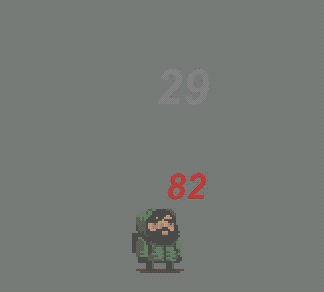``draw_text_transformed_color(x,y,string,xscale,yscale,angle,c1,c2,c3,c4,alpha)``

• x,y——文字绘制坐标
• string——需要绘制的文字内容
• xscale,yscale——文字的横纵向缩放比
• angle——文字绘制角度
• c1,c2,c3,c4——左上左下右上右下四个顶点的颜色值，中间的颜色会自动过渡
• alpha——透明度

## 实现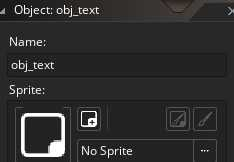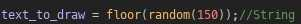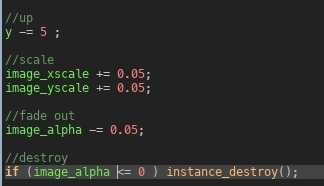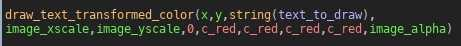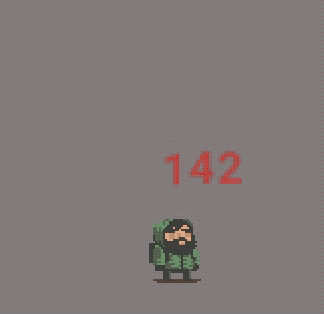## 优化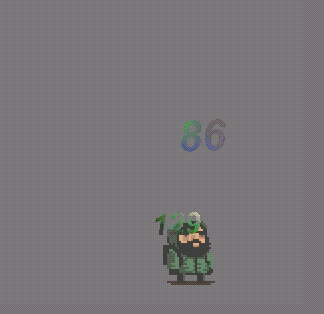``````//Create事件
//随机获取四个顶点的颜色
color = [c_green,c_white,c_red,c_purple,c_yellow,c_blue,c_lime,c_maroon,c_orange]
color1_to_draw = color[random(7)];//c1
color2_to_draw = color[random(7)];//c2
color3_to_draw = color[random(7)];//c3
color4_to_draw = color[random(7)];//c4
//随机设定显示的数字
text_to_draw = floor(random(150));//String``````
``````//Step事件
//up
y -= 5 ;

//scale
image_xscale += 0.05;
image_yscale += 0.05;

image_alpha -= 0.05;

//destroy
if (image_alpha <= 0 ) instance_destroy();``````
``````//Draw事件
//设定自定义字体，这个字体的字号我设置了100
draw_set_font(fnt_test);
//文字居中排版
draw_set_halign(fa_center)
//绘制文字，角度用了wave脚本，这个脚本以前介绍过
draw_text_transformed_color(x,y,string(text_to_draw),image_xscale/10,image_yscale/10,wave(-10,10,5,0),color1_to_draw,color2_to_draw,color3_to_draw,color4_to_draw,image_alpha)``````

## 示例下载

（转发自：原日志地址
##### [ 分享 ]在GMS2中实现动态遮罩效果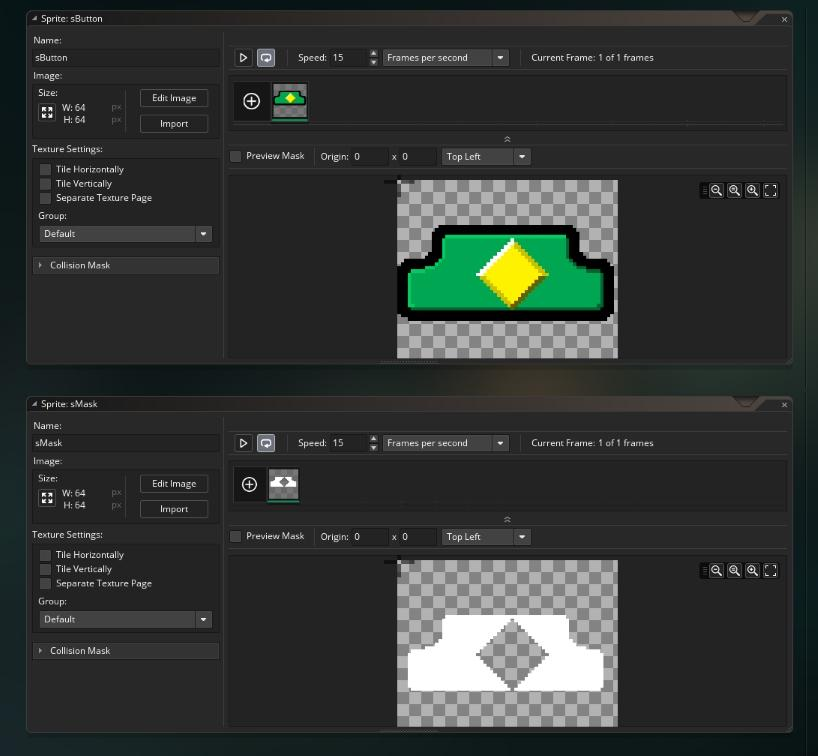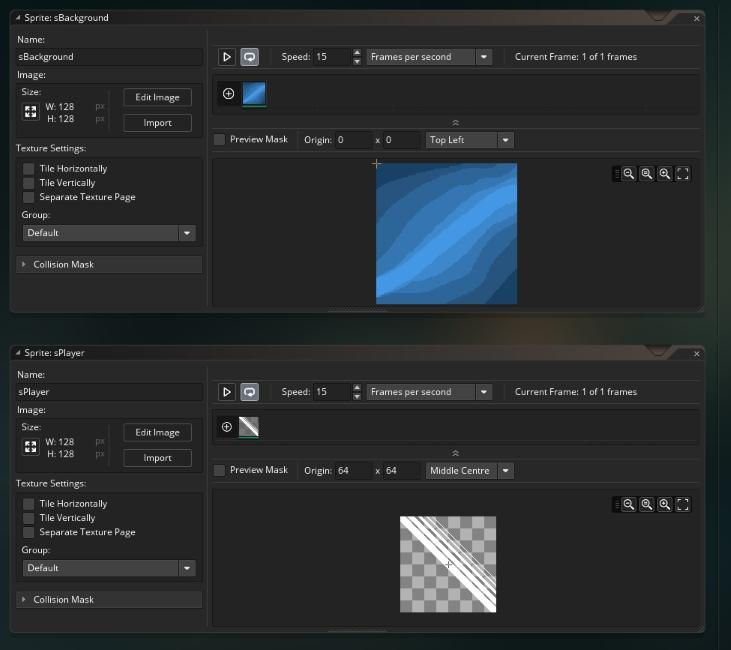``````draw_sprite(sBackground,0, 0,0);
draw_sprite_ext(sButton,0, 32+4,32+4,1,1,0,c_black,0.5);
draw_sprite(sButton,0, 32,32);``````

``````gpu_set_blendenable(false)
gpu_set_colorwriteenable(false,false,false,true);
draw_set_alpha(0);
draw_rectangle(0,0, room_width,room_height, false);``````

``````draw_set_alpha(1);
gpu_set_blendenable(true);
gpu_set_colorwriteenable(true,true,true,true);``````

``````gpu_set_blendmode_ext(bm_dest_alpha,bm_inv_dest_alpha);
gpu_set_alphatestenable(true);
draw_sprite(sPlayer,0, mouse_x,mouse_y);
gpu_set_alphatestenable(false);
gpu_set_blendmode(bm_normal);``````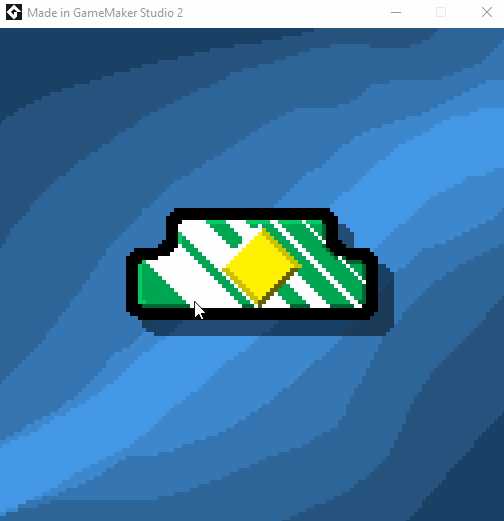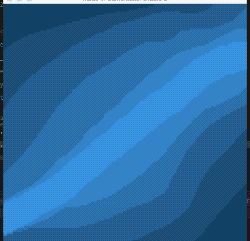（转发自：原日志地址
##### 月度挑战——多人游戏（本地）

————————————————————————

• 在你正在做的游戏里实现这个功能
• 做一个小的Demo演示
• 把相关的代码直接发出来
• 任何方式，你喜欢就好！

##### 在GMS2中配置iOS设备调试

GameMaker Studio 2的移动版可以支持iOS和Android双平台的导出，之前我曾经写过Android平台的真机调试的配置方式，前阵子有朋友在后台问我怎么导出iOS版本，因此今天就来介绍一下。

# 概述VM模式是通过一个叫“YOYO Runner”的特殊运行器直接运行你的代码，而YYC模式则是完全编译成对应平台的原生代码再执行，前者可以理解成是使用GMS2自带的一个万能模拟器来运行你的游戏。

VM模式的运行效率稍微低一些，但是编译速度更快，并且可以使用调试模式（Debugger），而在YYC模式下是无法调试游戏的，当然YYC模式的运行效率更高性能更可靠，不过如果游戏比较大逻辑比较复杂的话编译的时间可能会比较长，各位可以自行权衡取舍。

# 设备配置# 平台设置# 测试项目（转发自：原日志地址
##### 游戏暂停的简单实现（2/2）

HeartBeast的教程已经传到B站了——简单实现游戏暂停

## 控制暂停对象接着我们创建一个“Create”事件，并在其中定义两个全局变量：

``````global.paused_ = false ;
global.screen_pic = noone;``````

• paused_：标识当前是否暂停状态
• screen_pic：用来保存暂停前的游戏画面``````if !global.paused_{
global.paused_ = true
if sprite_exists(global.screen_pic) sprite_delete(global.screen_pic) ;
global.screen_pic = sprite_create_from_surface(application_surface,0,0,room_width,room_height,false,false,0,0)

instance_deactivate_all(all)
instance_create_layer(room_width/2,room_height/2,"pipe",obj_continue)
}``````

``if not global.paused_``

``if sprite_exists(global.screen_pic) sprite_delete(global.screen_pic) ;``

``global.screen_pic = sprite_create_from_surface(application_surface,0,0,room_width,room_height,false,false,0,0)``

``````instance_deactivate_all(all)
instance_create_layer(room_width/2,room_height/2,"pipe",obj_continue)``````

## 恢复游戏对象``````instance_activate_all();
global.paused_ = false;
instance_destroy();``````

``````draw_sprite(global.screen_pic,0,0,0)
draw_self()``````

HB的教学视频中使用了用户事件来封装暂停的操作，而其实也可以用脚本来单独封装，HB没有使用脚本的原因是他的教学视频采用了试用版，对脚本的数量有限制。

（转发自：原日志地址

## 前言

GMS2每次版本更新时都会卡住很久，而且因为更新界面比较简陋，用户无法通过界面信息及时了解到更新究竟是卡在了哪里，只能干瞪眼，让人无名火起。

## 操作

• step1

https://account.yoyogames.com/runtimes

• step2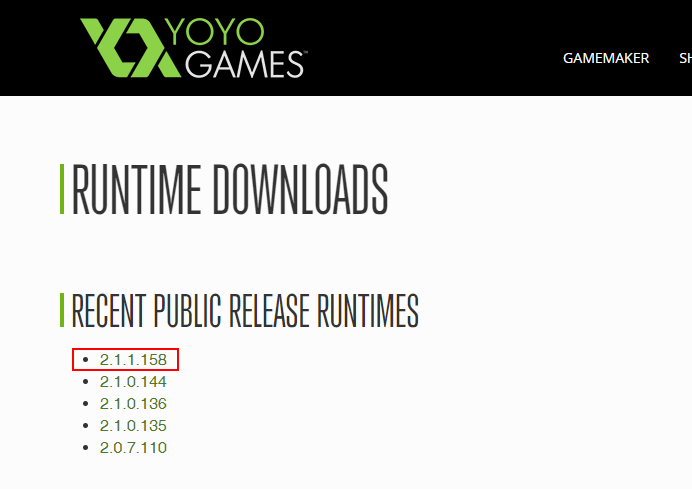• step3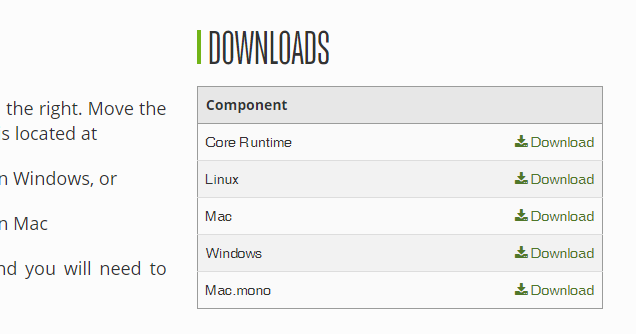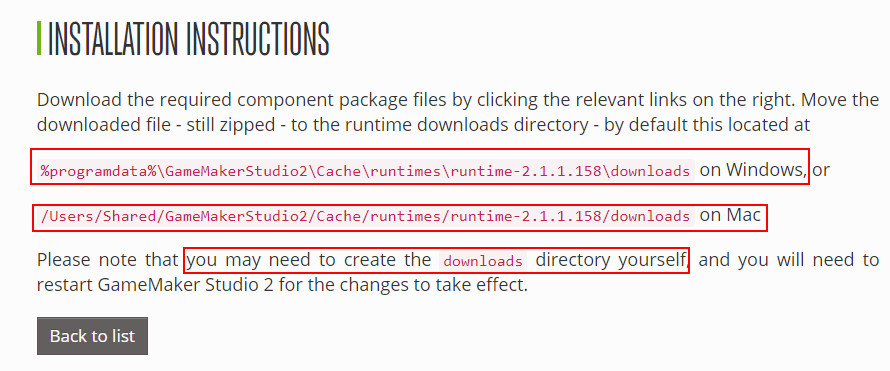• step4

（请留意版本号，其中后缀为zip的文档即是runtime文件）（转发自：原日志地址
##### [ 开发 ] Reddit的GMS十月挑战——鬼怪特效

• 直接在你正在制作的项目中使用该功能
• 制作一个全新的demo
• 提交一份相关的代码
• 以及其他任何你喜欢的形式！##### 游戏暂停的实现方式(1/2)

Flappy Bird的暂停界面属于现在手机游戏特别典型那种，当玩家按下暂停按钮以后在当前的画面至上浮出一个暂停菜单，菜单里一般有“继续”，“重新开始”，“退出游戏”等一些基本的选项操作，如果玩家按下“继续”那就要回到游戏界面可以继续操作。

• 制作一个专门的“暂停菜单”的游戏场景（room），当用户点击暂停以后跳转到那个场景
• 在用户按下暂停按钮的同时把当前画面截屏并设置为背景画面，同时把场景中所有的对象全部禁用（deactivate），然后创建一个新的暂停菜单的对象供用户操作``````room_persistent = true;     //把持久化属性设置为true
room_goto(room_pause);  //跳转去暂停菜单场景
global.current_room = room //把当前场景保存到一个全局变量中``````

``room_goto(global.current_room);   //返回暂停前的游戏场景``

（转发自：原日志地址
##### 在GMS中使用DS MAP存储/读取游戏信息

• ds_map_secure_save• ds_map_exists
• ds_map_create
• ds_map_clear
• ds_map_copy
• ds_map_replace
• ds_map_delete
• ds_map_empty
• ds_map_size
• ds_map_find_first
• ds_map_find_last
• ds_map_find_next
• ds_map_find_previous
• ds_map_find_value
• ds_map_write
• ds_map_destroy

``````save_1 = ds_map_create(); //创建一个ds_map保存到save_1变量中

//

ds_map_secure_save(save_1, "save1.dat" ) ;//把save_1中的ds_map保存为一个名为"save1.dat"的物理文件
ds_map_destroy(save1.dat);//释放内存``````

``````load_save = ds_map_secure_load("save1.dat")//加载"save1.dat"文件中的ds_map保存到"load_save"中

//加载ds_map中的数据保存到游戏变量中

• 加密性更好
• ds_map的数据结构也更好与ds_list和ds_grid等联动
• ini配置文件同时只能开启一个，ds_map只要内存不炸理论上可以多个同时操作

（转发自：原日志地址
##### GameMaker Studio 2中简易存/读档

• 自带函数game_save()
• ini配置文件保存
• ds_map/ds_list存储

## 自带函数game_save()

• game_save()

``````game_save("save.dat")

## ini配置文件保存``````ini_open("saveData.ini"); //打开ini文件，如果不存在则新建
ini_write_real("Variables", "score", score); //第一个值是section，第二个值是key，第三个值是对应要写入的value
ini_write_string("Variables","string",string); //同上，不过这是写入字符串的方法
ini_close(); //用完记得关闭文件否则容易内存溢出``````

``````ini_open("saveData.ini");
ini_close();``````

（转发自：原日志地址

## Wrap()

Wrap这个脚本是用来做什么的呢？先来看一下这个GIF图其实就是把传入的一个值约束在指定的范围内来回递增/减，并且钟摆循环。### 代码正文

``````///@description Wrap(value,min,max)
///@function Wrap
///@param value
///@param min
///@param max
//returns the value wrapped

var _val = argument;
var _max = argument;
var _min = argument;

if(_val mod 1==0 )
{
while(_val > _max) || _val < _min)
{
if(_val > _max)
{
_val = _min + _val - _max -1 ;
}
else if (_val < _min)
{
_val = _max + _val - _min + 1 ;
}
else
_val = _val ;
}
return(_val);
}
else
{
var _old = argument + 1 ;
while(_val != _old)
{
_old = _val ;
if (_val < _min)
_val = _max - (_min - _val) ;
else if(_val > _max)
_val = _min + (_val - _max) ;
else
_val = _val ;
}
return(_val);
}``````

## JumpInDirection()

### 代码正文

``````/// @description JumpInDirection(length,direction)
/// @param length
/// @param direction
var len = argument0 ;
var dir = argument1;

x += lengthdir_x(len,dir);
y += lengthdir_y(len,dir);``````

## Chance()

``````if Chance(0.7)
{
//写在这里的代码就有70%的概率被触发
}``````

### 代码正文

``return argument0 > random(1) ;``
（转发自：原日志地址
##### Reddit的GMS九月挑战——系统时间

• 直接在你正在制作的项目中使用该功能
• 制作一个全新的demo
• 提交一份相关的代码
• 以及其他任何你喜欢的形式！

• current_second
• current_minute
• current_hour
• date_current_datetime
• date_get_second
• date_get_minute
• date_get_hour